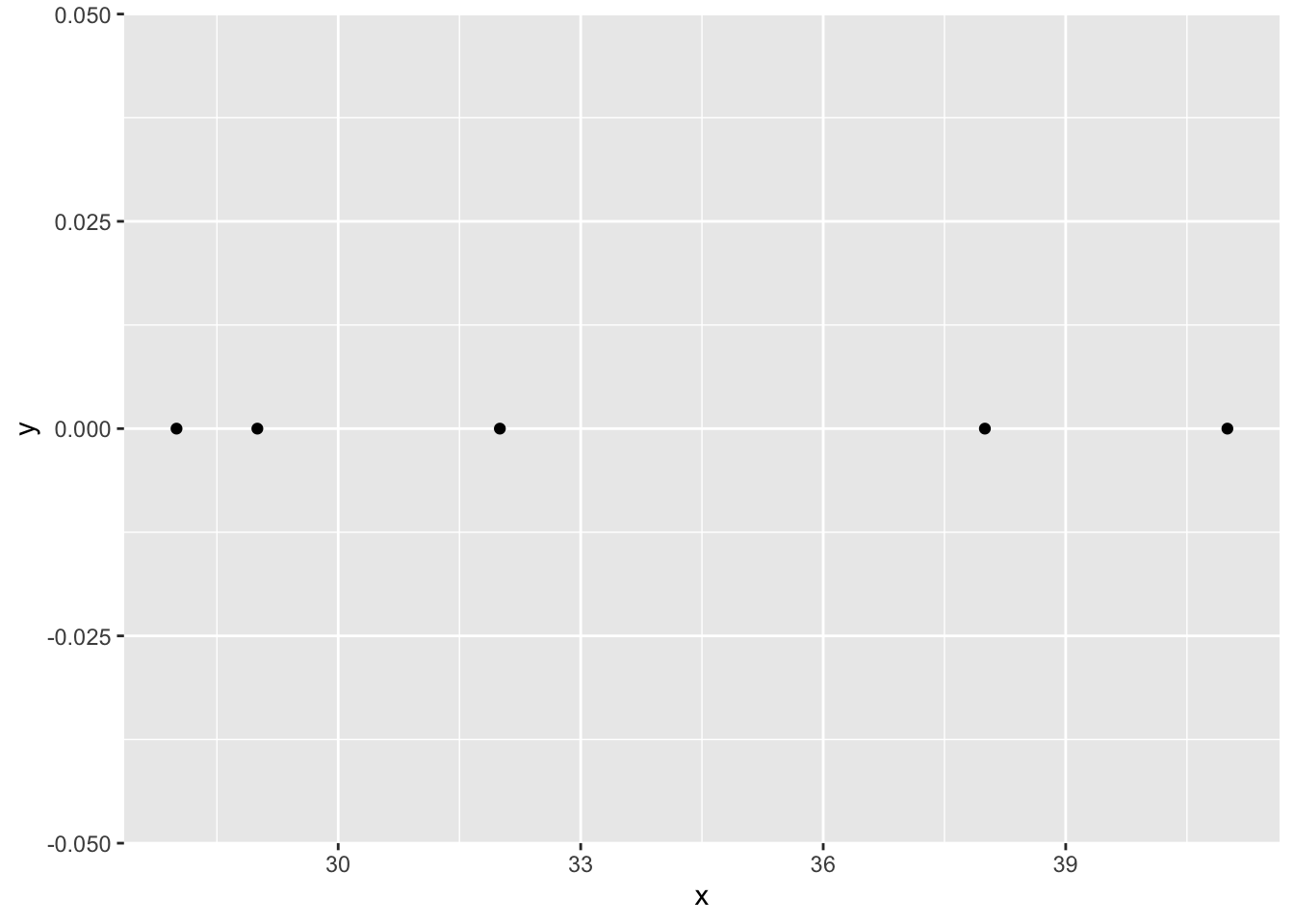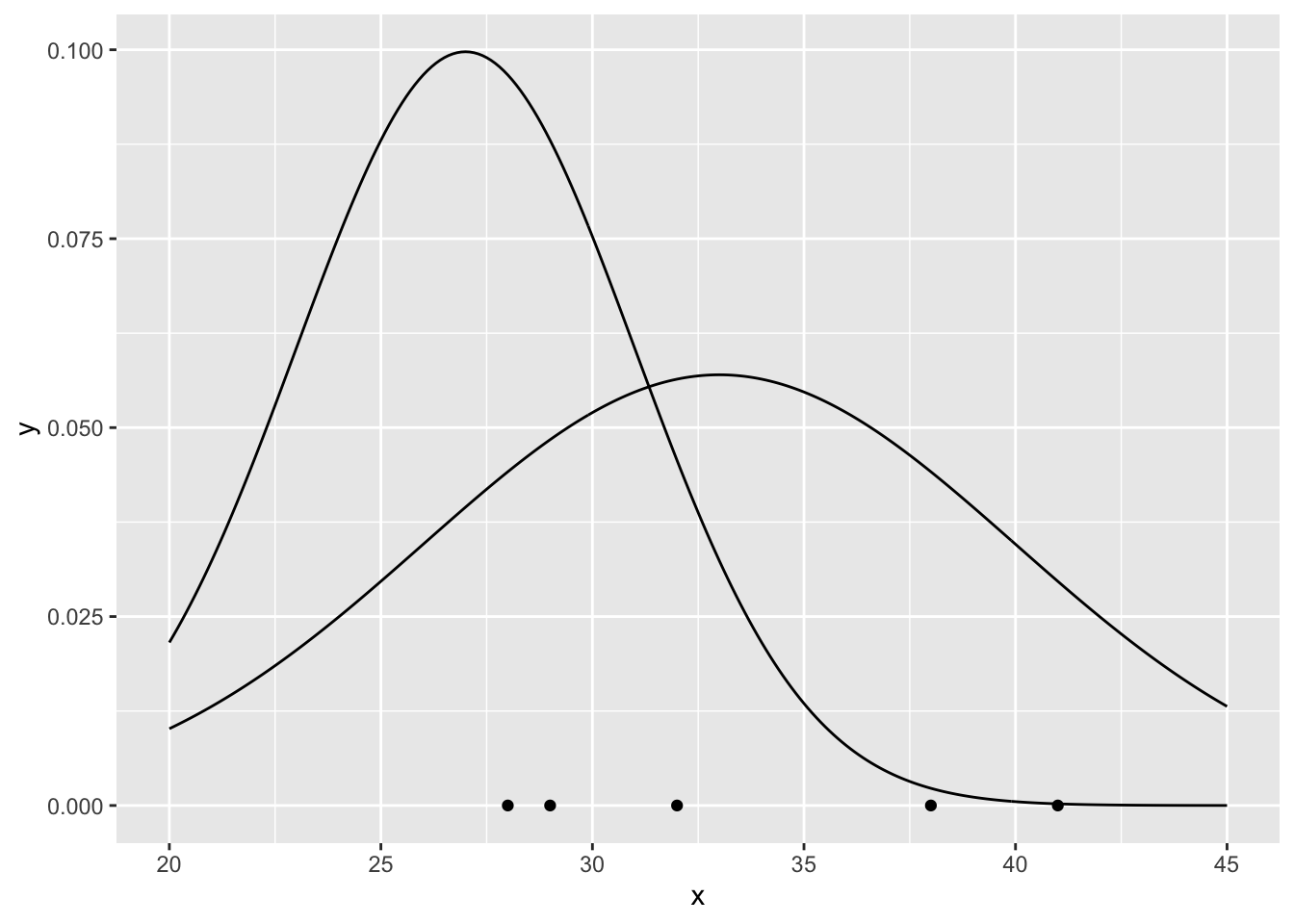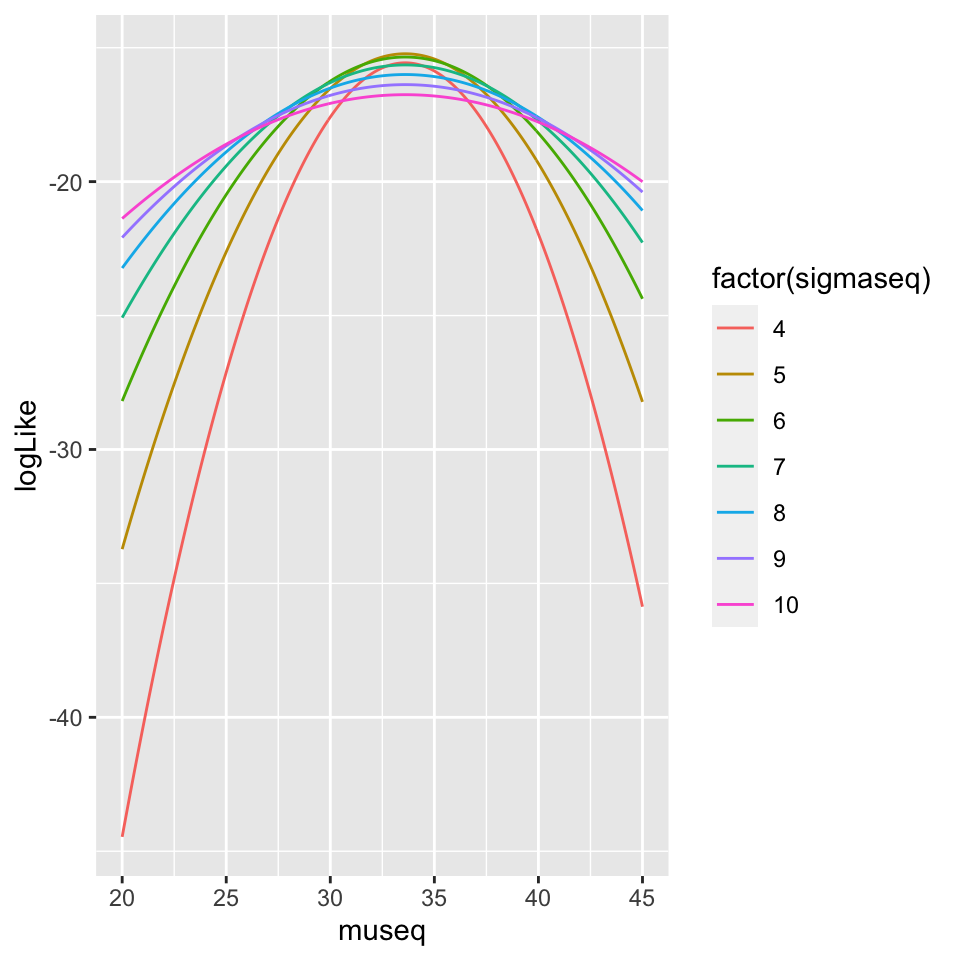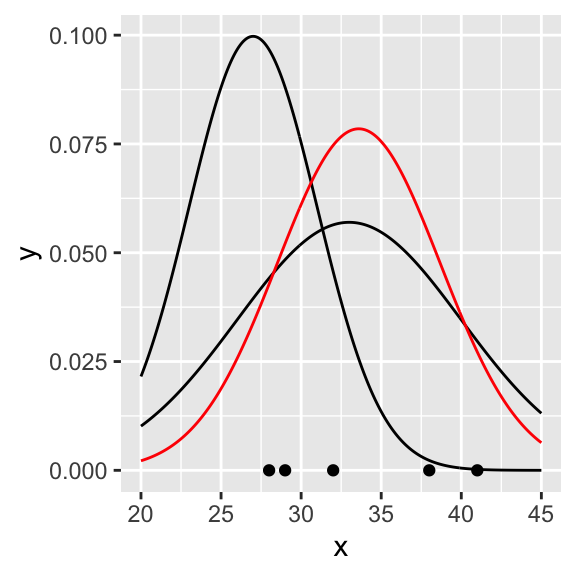This is an example to illustrate MLE. Actually, it could be easy demonstrated that when the parametric family is the normal density function, then the MLE of $$\mu$$ is the mean of the observations and the MLE of $$\sigma$$ is the uncorrected standard deviation of the observations.

Suppose that we have the following independent observations and we know that they come from the same probability density function

library(tidyverse)

x<- c(29, 41, 32, 28, 38) #our observations

dat <- tibble(x, y=0) #we plotted our observations in the x-axis
p <- ggplot(data = dat, aes(x = x, y = y)) + geom_point()
pWe don’t know the exact probability density function, but we know that the shape (the parametric family) is the shape of the normal density function $f(x;\theta)=f(x;\mu,\sigma)=\frac{1}{\sqrt{2\pi\sigma^2}}e^{-\frac{(x-\mu)^2}{2\sigma^2}}$ Let’s plot two arbitrary probability density functions fixing the parameters of the parametric family

xseq <- seq(20, 45, .1)
f1 <- dnorm(xseq, 33, 7) #mu=33, sigma=7
f2 <- dnorm(xseq, 27, 4) #mu=27, sigma=4
curve1 <-tibble(xseq,f1)
curve2 <-tibble(xseq,f2)

p <- p +
geom_line(data = curve1, aes(x = xseq, y = f1)) +
geom_line(data = curve2, aes(x = xseq, y = f2))
pIt is clear that the probability density function $$f$$ for $$\mu=33$$ and $$\sigma=7$$ describes the observations better than the other one. We are interested in the $$f$$ that maximizes $$L$$.

The joint density function is $f(x;\mu,\sigma)=f(x_1;\mu,\sigma)f(x_2;\mu,\sigma)...f(x_5;\mu,\sigma)=$ $=\frac{1}{\sqrt{2\pi\sigma^2}}e^{-\frac{(x_1-\mu)^2}{2\sigma^2}}\frac{1}{\sqrt{2\pi\sigma^2}}e^{-\frac{(x_2-\mu)^2}{2\sigma^2}}...\frac{1}{\sqrt{2\pi\sigma^2}}e^{-\frac{(x_5-\mu)^2}{2\sigma^2}}.$ that when considered as a function of the parameters is $L(\mu,\sigma|x)=L(\mu,\sigma|29,41,32,28,38)=f(x|\mu,\sigma)=f(29,41,32,28,38|\mu,\sigma)=$ $=f(29|\mu,\sigma)f(41|\mu,\sigma)...f(38|\mu,\sigma)=$ $=\frac{1}{\sqrt{2\pi\sigma^2}}e^{-\frac{(29-\mu)^2}{2\sigma^2}}\frac{1}{\sqrt{2\pi\sigma^2}}e^{-\frac{(41-\mu)^2}{2\sigma^2}}...\frac{1}{\sqrt{2\pi\sigma^2}}e^{-\frac{(38-\mu)^2}{2\sigma^2}}$

and $log(L)=log(f(x;\mu,\sigma))=log(f(x_1;\mu,\sigma))+log(f(x_2;\mu,\sigma))+...+log(f(x_5;\mu,\sigma))=$ $=log(f(29;\mu,\sigma))+log(f(41;\mu,\sigma))+...+log(f(38;\mu,\sigma))$

logL <- function(p) sum(log(dnorm(x, p, p))) # p = c(p, p) = c(mu, sigma)
#logL <-function(p) sum(dnorm(x, p, p,log = TRUE)) #alternative way to write it

We can calculate the $$log(L)$$ for the two previous examples to verify that $$log(L)$$ is larger for $$\mu=33$$ and $$\sigma=7$$

logL(c(33, 7))
##  -15.66098
logL(c(27, 4))
##  -22.36991
logL(c(33, 7)) > logL(c(27,4))
##  TRUE

Let’s plot the $$log(L)$$ for some values of $$\mu$$ and $$\sigma$$

dLogL <- crossing(museq = seq(20, 45, .1), sigmaseq = seq(4, 10, 1)) %>%
rowwise() %>%
mutate(logLike = logL(c(museq, sigmaseq)))

ggplot(data = dLogL,
aes(x= museq, y=logLike, color = factor(sigmaseq))) +
geom_line()So it seems that values of $$\mu$$ around 34 and $$\sigma$$ around 5 maximizes log(L). Let’s maximize it properly using optim. Because optim search for a minimum, we need to minimize $$-log(L)$$

negLogL <- function(p) -logL(p)
estPar <- optim(c(20, 10), negLogL) # some initial parameters
MLEparameters <- estPar\$par

MLEparameters
##  33.598814  5.083355

So, we found that from the parametric family, the probability density function that better characterizes the observations according to MLE is the one described by the parameters $$\mu=$$ 33.5988136 and $$\sigma=$$ 5.0833546. Let’s plot the distribution in red in the previous graph

fMLE <- dnorm(seq(20,45,.1), MLEparameters, MLEparameters)
curveMLE<-tibble(xseq, fMLE)

p + geom_line(data = curveMLE, aes(x = xseq, y = fMLE), color = "red")It could be easy demonstrated that actually when the parametric family is the normal density function, then the MLE of $$\mu$$ is the mean of the observations

mean(x)
##  33.6

and the MLE of $$\sigma$$ is the uncorrected standard deviation of the observations

sqrt(mean((x - mean(x))^2))
##  5.083306